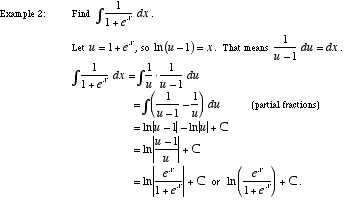index: click on a letter A B C D E F G H I J K L M N O P Q R S T U V W X Y Z A to Z index index: subject areas numbers & symbols sets, logic, proofs geometry algebra trigonometry advanced algebra & pre-calculus calculus advanced topics probability & statistics real world applications multimedia entrieswww.mathwords.com about mathwords website feedback

 Rationalizing Substitutions An integration method which is often useful when the integrand is a fraction including more than one kind of root, such as. A different type of rationalizing substitution can be used to work with integrands such as. Note: This method transforms the integrand into a rational function, hence the name rationalizing.See also